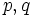# Homomorphism from Baer-Specker group to group of integers that is zero on restricted direct product is zero

## Statement

Denote by$G$ the Baer-Specker group, the external direct product of countably many copies of the group of integers. Denote by$H$ the subgroup that is the restricted direct product of the direct factors.

Suppose$\varphi: G \to \mathbb{Z}$ is a homomorphism of groups such that the restriction$\varphi|_H$ is the trivial map. Then,$\varphi$ is also the trivial map.

## Proof

Given:$G$ is the Baer-Specker group,$H$ is the restricted direct product of the direct factors.$\varphi: G \to \mathbb{Z}$ is a homomorphism such that the restriction$\varphi|_H$ is the trivial map.

To prove:$\varphi(u) = 0$ for all$u \in G$.

Proof:

Step no. Assertion/construction Facts used Given data Previous steps used Construction
1 Suppose$p$ is a prime number. Then, for any element of$G$ of the form$g = (a_1p, a_2p^2, \dots, a_np^n, \dots)$, with$a_i \in \mathbb{Z}$,$\varphi(g) = 0$$\varphi$ is a homomorphism and$\varphi|_H = 0$ [SHOW MORE]
2 Pick two distinct primes$p, q$. Every element of$G$ can be expressed as the sum of elements$g = (a_1p, a_2p^2, \dots, a_np^n, \dots)$ and$h = (b_1q, b_2q^2, \dots, b_nq^n, \dots)$ where all the$a_i$ and$b_i$ values are integers. This follows from the Chinese remainder theorem applied in each coordinate.
3$\varphi(u) = 0$ for any$u \in G$$\varphi$ is a homomorphism Steps (1), (2) Express$u = g + h$ as per Step (2), then use Step (1) to show that$\varphi(g) = \varphi(h) = 0$. Thus,$\varphi(u) = 0$.